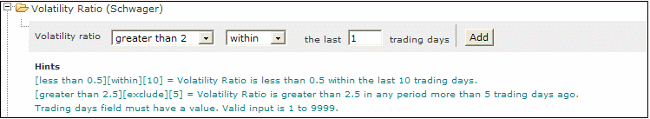# Stock Screener

## Volatility Ratio (Schwager)

• The Volatility Ratio identifies days with exceptionally wide trading ranges (the distance between High and Low) and is used to signal likely reversal days.
• Details of the indicator can be found at Volatility Ratio (Schwager).
• This stock screen combines well with Volume Spikes.

### Signals

• Volatility Ratio (Schwager) > 2.0
The indicator gives a reading greater than 2 when the true range is more than double its 14-day exponential moving average.To Set the Volatility Ratio screen:

1. Select the Volatility Ratio (Schwager) filter
2. Select the amount the Volatility Ratio would be greater or less than, using the drop down menu
3. Enter the number of days within which the signal must have occurred or excluded